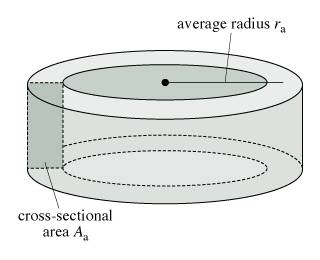# An Air-Filled Toroidal Solenoid

## Homework StatementAn air-filled toroidal solenoid has a mean radius of 14.5 cm and a cross-sectional area of 4.99 cm^2 (see the figure). The current flowing through it is 11.7 A, and it is desired that the energy stored within the solenoid be at least 0.388 J.

What is the least number of turns that the winding must have?
Express your answer numerically, as a whole number, to three significant figures.

## Homework Equations

$$B = \frac {\mu_0 N I}{2 \Pi r}$$

$$\Phi_B = \oint \vec{B} \cdot \vec{dA}$$

$$U = \frac {1}{2} L I^2$$

$$L = \frac {N \Phi_B}{i}$$

## The Attempt at a Solution

Solved for N to get the Number of Turns.

$$N = \sqrt{\frac {4 (\Pi) U r}{\mu_0 I^2 A}}$$

$$N = \sqrt{\frac {(4) (\Pi) (0.388j) (0.145m)}{(4 (\Pi) (10^{-7}) \frac{wb}{Am}) (11.7A^2) (0.0499m^2)}}$$

N = 287 turns

Do I have some conversion wrong or did I miss something.

Last edited:

Found my error in my conversion of $$cm^2$$ to $$m^2$$.
I did my the correct conversion is $$\frac{4.99}{10000}$$